## Heat Conduction Equation--Disk

To solve the Heat Conduction Equation on a 2-D disk of radius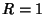, try to separate the equation using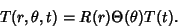(1)

Writing the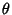and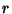terms of the Laplacian in Spherical Coordinates gives(2)

so the Heat Conduction Equation becomes(3)

Multiplying through bygives(4)

Theterm can be separated.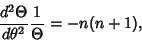(5)

which has a solution(6)

The remaining portion becomes(7)

Dividing by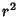gives(8)

where a Negative separation constant has been chosen so that the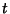portion remains finite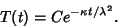(9)(10)(11)

which is the Spherical Bessel Differential Equation. If the initial temperature is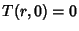and the boundary condition is, the solution is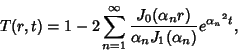(12)

whereis theth Positive zero of the Bessel Function of the First Kind.